Courses

# Test: Absolute Equations- 1

## 15 Questions MCQ Test Quantitative Aptitude for SSC CGL | Test: Absolute Equations- 1

Description
This mock test of Test: Absolute Equations- 1 for GMAT helps you for every GMAT entrance exam. This contains 15 Multiple Choice Questions for GMAT Test: Absolute Equations- 1 (mcq) to study with solutions a complete question bank. The solved questions answers in this Test: Absolute Equations- 1 quiz give you a good mix of easy questions and tough questions. GMAT students definitely take this Test: Absolute Equations- 1 exercise for a better result in the exam. You can find other Test: Absolute Equations- 1 extra questions, long questions & short questions for GMAT on EduRev as well by searching above.
QUESTION: 1

### Select the correct algebraic expression for the following number line representation: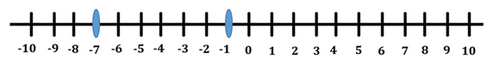Solution:

We have to find the expression |x – a| = b for which the solution set is -7 and -1.

We already know that the two points will be symmetrical about a.

So a is the mid-point of these two points.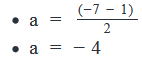ow, b is simply the distance between a and either of the points.

As we can see from the given figure, (also as we already know),

Distance between -4 and -1 (or -7) is simply 3 units.

b = 3

Therefore the required expression is: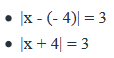QUESTION: 2

### What is the value of x? (1)  |2x + 3| = 4 (2)  |4x – 4| = 2

Solution:

Steps 1 & 2: Understand Question and Draw Inferences

We need to find the value of x from the given two statements.

Step 3: Analyze Statement 1

Statement I says |2x + 3| = 4.

Now let’s convert this into the standard form by dividing with 2 on both sides.

• |x + 3/2| = 2
• |x + 1.5| = 2
• |x – (-1.5)| = 2

So x is at a distance of 2 units from the point -1.5 on the number line. If we plot the possible points on number line, it looks something like the below figure.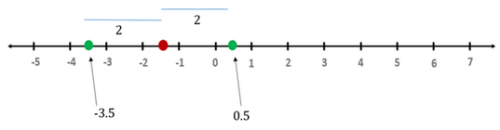We can see that there are two possible values for x: -3.5 and 0.5.

Therefore Statement 1 is not sufficient to arrive at a unique answer.

Step 4: Analyze Statement 2

Now let’s look at statement 2. Statement 2 says |4x – 4| = 2

Now let’s convert this into the standard form by dividing with 4 on both sides.

• |x – 1| = 2/4
• |x – 1| = ½ = 0.5

Hence, x can be 0.5 or 1.5.

Therefore Statement 2 is not sufficient to arrive at a unique answer.

Step 5: Analyze Both Statements Together (if needed)

Analyzing both statements together, we find that x must satisfy the possibilities represented by both the statements. Only x = 0.5 satisfies both statements and gives us a unique answer.

Statement 1 and statement 2 together are sufficient to arrive at a unique answer.

QUESTION: 3

### Select the correct algebraic expression for the following number line representation: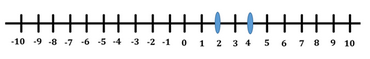Solution:

We have to find the expression |x – a| = b for which the solution set is 2 and 4.

We already know that the two points will be symmetrical about a.

So a is the mid-point of these two points.

• a=(2+4)2
•
• a=3
•

Now, b is simply the distance between a and either of the points.

As we can see from the given figure, (also as we already know),

Distance between 3 and 4 (or 2) is simply 1 unit.

b = 1

Therefore the required expression is:

|x -3| = 1

QUESTION: 4

Select the possible values of x if |x-4| = 6.

Solution:

So x is at a distance of 6 units from the point 4 on the number line. If we plot the possible points on number line, it looks something like the below figure.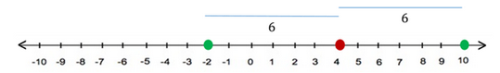Therefore there are two possible values for x: -2 and 10. (As shown in the above figure).

QUESTION: 5

Select the correct algebraic expression for the following number line representation: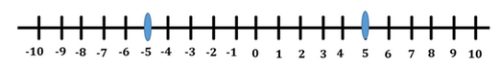Solution:

We have to find the expression |x – a| = b for which the solution set is -5 and 5.

We already know that the two points will be symmetrical about a.

So a is the mid-point of these two points.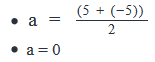Now, b is simply the distance between a and either of the points.

As we can see from the given figure, (also as we already know),

Distance between 0 and 5 (or -5) is simply 5 units.

b = 5

Therefore the required expression is:

|x - 0| = 5

|x| = 5

QUESTION: 6

If x is a positive integer and |6 – 3x| = 9, what is the value of x?

Solution:

Given absolute value equation is:

|6 - 3x| = 9

Let us convert this into standard form.

First let us make the coefficient of x positive.

We know that

(6 - 3x) = -(3x - 6)

We also know that

|-x| = |x|

Therefore

|3x - 6| = 9

Now let us divide both sides by the coefficient of x, i.e., 3.

We get:

|x - 2| = 3

So x is at a distance of 3 units from the point 2 on the number line.

If we plot the possible points on number line, it looks something like the below figure.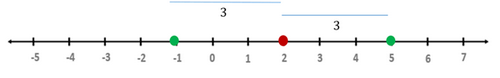From the above figure, it is clear that the possible values of x are -1 and 5.

However, it is given that x is a positive integer.

Therefore the only possible value for x is 5.

QUESTION: 7

Select the possible values of x if |x+4| = 6

Solution:

The given expression can be written as:

|x - (-4)| = 6

So x is at a distance of 6 units from the point -4 on the number line. If we plot the possible points on number line, it looks something like the below figure.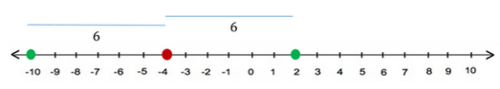Therefore there are 2 possible values for x: -10 and 2 (As shown in the above figure).

QUESTION: 8

If -|x+1| = b, where b is a non-zero integer, which of the following statements must be true?

I.  b < 0

II.  x < -b

III.  x > b

Solution:

Given, -|x+1| = b

• |x+1| = -b
• |x – (-1)| = -b

Since the left hand side (modulus) is never negative, we can infer that “-b” on the RHS is positive. (It is already given that b is non-zero).

• -b > 0
• b < 0

Therefore statement I is correct.

Now let us try to represent the given expression on number line and see what it says.

|x – (-1)| = -b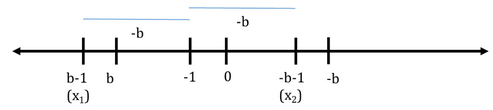There are two possible values of x (x1, x2) that are at a distance of “-b” from -1. (As shown in the above figure.)

You can see that both these values are to the left side of “-b”.

Therefore both of them are less than “-b”

So statement II is correct.

Also, you can see from the above figure that only one of the possible values of x is greater than b while the other is lesser than b. Therefore statement III is incorrect.

QUESTION: 9

Which of the following inequalities is an algebraic expression for the shaded part of the number line above?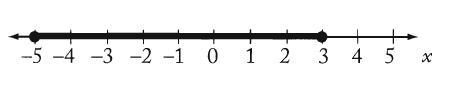Solution:

The length of the line is 8. Middle point = 8/2 = 4. The point on the number line equidistant at a length of 4 from each extremeties (-5 and 3) is -1. So, the equation turns out to be,

|x - (equidistant point)| <= Middle Point
i.e. |x-(-1)| <= 4
i.e. |x+1| <= 4

Ans - (B)

QUESTION: 10

If |x| > 6, which of the following expressions must be correct?

1.x < 6

II.–x < 6

III. x < -6 OR x > 6

Solution:

Please note that the question stem uses the word “must be true”. Hence, we need to reject the values that can be true but are not “must be true”.

We know that |x – a| > b represents those numbers on the number line that are at a distance greater than b units from a.

So |x| > 6 represents those numbers on the number line that are at a distance greater than 6 from 0.

If we represent this on a number line: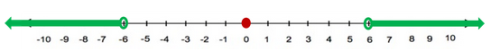So, as you can see, the |x| > 6 essentially represents the set of values of x that satisfy x > 6 or x < -6

Therefore only statement III is correct.

Note: As a thumb of rule, you can also remember the following:

If |x – a| > b, then (x – a) > b or (x – a) < -b

QUESTION: 11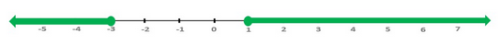Which of the following inequalities is an algebraic expression for the shaded part of the number line above?

Solution:

Step 1: Question statement and Inferences

This is a Reverse Logic Inequality. Here, we are given the solution set of the inequality, and we are asked to determine the expression for the inequality. So, we will follow the process below to solve it:

Step 1: Plot the mid-point of the solution set on the number line. Mid-point = a

Step 2: Find the distance of either end-point from the mid-point. This distance =b

Step 3: Insert the appropriate sign of inequality between |x-a| and b

Step 2 & 3: Working with the answer choices and calculating the final answer

Let’s apply this process to the current problem:

Step 1: The mid-point of the solution set is -1. Thus, a = -1

Step 2: The distance of -3 from -1 = the distance of 1 from -1 = 2 units. Thus, b = 2

Step 3: Since points -3 and 1 are also included in the solution set (as indicated by the full circles at these two points), the correct inequality sign will be ≥ and not just >

So, the expression for the inequality is:

|x – (-1)| ≥ 2

That is, |x+1| ≥ 2

QUESTION: 12

If x is an integer where 2 -|x – 3| < 2, how many values of x are possible?

Solution:

Step 1: Question statement and Inferences

Given the inequality and that x is an integer, x may have a limited number of possibilities:

2 - |x - 3| < 2

Step 2 & 3: Simplifying the fraction and calculating the final answer

Find out by simplifying the inequality. Start by subtracting 2 from both sides:

- |x - 3| < 0

Multiply both sides by –1 so the absolute value expression becomes positive, which also switches the inequality:

|x - 3| > 0

Any value of x other than 3 results in a number inside the absolute value bars, the absolute value of which is greater than zero. x can be any number other than 3, so the number of values is infinite.

QUESTION: 13

If x is a positive number and |-4x + 8| ≥ 2, which of the following statements is correct?

Solution:

We know that |-4x + 8| = |-(4x -8)| = |4x -8|

Therefore we’re essentially given

|4x−8|≥2

Dividing both sides by 4, we get: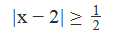Representing this on the number line: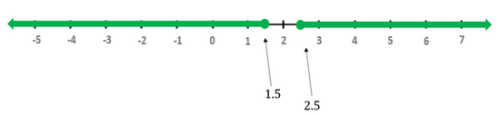Therefore we have

x ≥ 2.5 or x ≤ 1.5

QUESTION: 14

If |-1-x| ≤ 3, where x is a positive integer, what is the smallest possible value of x?

Solution:

We know that |-1 – x| = |-(1 + x)| = |1 + x|

Therefore we’re essentially given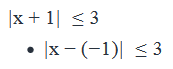Representing this on the number line we have: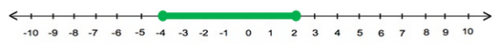So we can see that

−4≤x≤2

However, it is given that x is a positive integer.

Therefore x > 0

Therefore we have

0<x≤2

Therefore the smallest possible value of x is 1.

QUESTION: 15

If x > 0, how many integer values of (x, y) will satisfy the equation 5x + 4|y| = 55?

Solution:

5x + 4|y| = 55
The equation can be rewritten as 4|y| = 55 - 5x.
Because |y| is non-negative, 4|y| will be non-negative. Therefore, (55 - 5x) cannot take negative values.

Because x and y are integers, 4|y| will be a multiple of 4.
Therefore, (55 - 5x) will also be a multiple of 4.
55 is a multiple of 5. 5x is a multiple of 5 for integer x. So, 55 - 5x will always be a multiple of 5 for any integer value of x.
So, 55 - 5x will be a multiple of 4 and 5.
i.e., 55 - 5x will be a multiple of 20.

Integer values of x > 0 that will satisfy the condition that (55 - 5x) is a multiple of 20:
1. x = 3, 55 - 5x = 55 - 15 = 40.
2. x = 7, 55 - 5x = 55 - 35 = 20
3. x = 11, 55 - 5x = 55 - 55 = 0.
When x = 15, (55 - 5x) = (55 - 75) = -20. Because (55 - 5x) has to non-negative x = 15 or values greater than 15 are not possible.
So, x can take only 3 values viz., 3, 7, and 11.

We have 3 possible values for 55 - 5x. So, we will have these 3 values possible for 4|y|.
Possibility 1: 4|y| = 40 or |y| = 10. So, y = 10 or -10.
Possibility 2: 4|y| = 20 or |y| = 5. So, y = 5 or -5.
Possibility 3: 4|y| = 0 or |y| = 0. So, y = 0.

Number of values possible for y = 5.

The correct choice is (C) and the correct answer is 5.